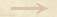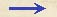Other Propositions projectile impetus Other Propositionprojectile impetusThe compounding of Impetus Galileo explored on several folio pages of Ms. Gal. 72 'what happens when a body is urged by a motion compounded of one which is horizontal and uniform and of another which is vertical but naturally accelerated; from these two components results the path of a projectile, which is a parabola. The problem is to determine the speed [impeto] of the projectile at each point.' (Discorsi, before Proposition 3, Theorem 3 of Book 3 of De motu locali) He considered, in particular, a composition rule according to which the horizontal impetus is just added to the vertical one like a number and later found the composition rule as formulated in 3/04-pr-01, according to which the square of the compounded impetus is equal to the sum of the squares of the components. (See alsoDamerow et al. 1992.Other Propositions projectile impetus Other Propositionprojectile impetus